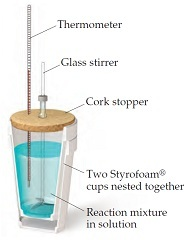# Problem: When a 4.25-g sample of solid ammonium nitrate dissolves in 60.0 g of water in a coffee-cup calorimeter, the temperature drops from 22.0 oC to 16.9 oC.You may want to reference (Pages 179 - 183) Section 5.5 while completing this problem.Assume that the specific heat of the solution is the same as that of pure water.Calculate delta(H) (in kJ/mol NH4NO3) for the solution proces.

###### FREE Expert Solution

Use the calorimetry equation to find the heat involved

Calculating the change in enthalpy for 1 mole ( MM of NH4NOis 80 g/mol)

96% (306 ratings)###### Problem Details

When a 4.25-g sample of solid ammonium nitrate dissolves in 60.0 g of water in a coffee-cup calorimeter, the temperature drops from 22.0 oC to 16.9 oC.You may want to reference (Pages 179 - 183) Section 5.5 while completing this problem.

Assume that the specific heat of the solution is the same as that of pure water.

Calculate delta(H) (in kJ/mol NH4NO3) for the solution proces.

Frequently Asked Questions

What scientific concept do you need to know in order to solve this problem?

Our tutors have indicated that to solve this problem you will need to apply the Constant-Pressure Calorimetry concept. You can view video lessons to learn Constant-Pressure Calorimetry. Or if you need more Constant-Pressure Calorimetry practice, you can also practice Constant-Pressure Calorimetry practice problems.